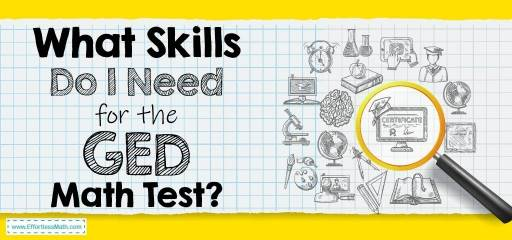# Math Skills You Need for the GED Math TestMany test-takers find it difficult to solve GED math questions every year. Despite the math anxiety, some test takers still make their way to success! Have you ever wondered why some test takers pass their GED Math test while some fail?

Students who pass the GED test have been found to have skills in topics related to the GED math questions. These math skills did not only help them to solve questions faster but also to arrive at the right answer.

With math skills and adequate preparation discussed in this article, you can overcome your phobia of failure and develop the courage to sit for your GED math exam.

So, are you eager to find out what skills I need for the GED math test? Don’t worry; this article will unveil the information on what other people are doing to succeed in their GED math test, together with what you can do to score above the cutoff mark.

The Absolute Best Book to Ace the GED Math Test

## GED Math Requirements

Students are requested to answer 46 questions, and you would need to score 145 points to attain the pass mark. However, Candidates are to attempt both sections in the GED math question.

The first part does not allow the candidate to use the calculator in answering all five questions. Note, in the first five questions, you would need skills in numbers and arithmetic.

The GED second part has an on-screen calculator that makes your work easier.

Altogether, test-takers are allowed to spend 1 hour 45 min on all questions.

Best GED Math Prep Resource

## What skills do I need to pass my GED Math Test?

Most students who have heard the news about the GED math test might want to find out how hard the GED test is and what skills they need to pass the GED test.

Here are the topics you will be dealing with and how to solve your GED math questions easily.

1. Number Operations – you would need to work on your numerical skills, including the ability to structure and organize. Understand the four basic operations, which are addition, subtraction, multiplication, and division.
2. Algebra, Functions, and patterns – understand fractions, percentile, probability, ratio, and proportion.
3. Measurement and Geometry – here, you will have to apply logical skills, visual skills, and measuring skills.
4. Statistics – understanding of statistical concepts and terms, analytical skills, and problem-solving skills.
5. Data analysis – understanding of formulas and their applications.
6. Probability you need to understand how to process information and the ability to organize structures. Know basic probability, including how to determine events and outcomes.

Conclusion

Most students are scared to take GED classes and the GED math test. However, if you carefully work according to the instructions given above on ‘what skills do I need for the GED math test’, success is assured.

After you’ve mastered all the vital skills you would need for your GED Math exam, including the GED math formulas, this is where self-evaluation comes in. Register for the GED math practice test to help you get familiar with the real GED math questions.

## High School Equivalency Tests

The Best Books to Ace the GED Math Test

## More from Effortless Math for GED Test …

Now that you’ve gone through the list of math concepts you need to master before test day, make sure you learned all GED Math topics by reviewing our Ultimate GED Math Course.

This course offers everything you will ever need to ace the GED Math test. Lessons, videos, worksheets, and practice tests are all available in this FREE GED Math course.

### Need to review the most common GED Math formulas?

We have prepared a complete list of GED Math formulas to help test takers practice and prepare for their GED Math test.

Don’t forget to take our Full-Length GED Math Practice Test and Free GED Math Practice Test. Taking a realistic and full-length GED Math test is the best way to measure your exam readiness.

The Perfect Prep Books for the GED Math Test

## Have any questions about the GED Test?

### What people say about "Math Skills You Need for the GED Math Test - Effortless Math: We Help Students Learn to LOVE Mathematics"?

No one replied yet.

X
49% OFF

Limited time only!

Save Over 49%

SAVE \$34

It was \$69.99 now it is \$35.99